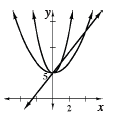### Home > CCA2 > Chapter 2 > Lesson 2.1.5 > Problem2-70

2-70.

Draw accurate graphs of $y=2x+5$, $y=2x^2+5$, $y=\frac{1}{2}x^2+5$ on the same set of axes. Label the intercepts.

1. In the equation $y=2x+5$, what does the $2$ tell you about the graph?

This is in the form $y=mx+b$. What does $m$ stand for?

2. Is the $2$ in $y=2x^2+5$ also the slope? Explain.Is this in the form, $y=mx+b$?

$2$ is the stretch factor. This is a curve, so it does not have a constant slope.